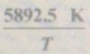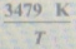# Problem: The vapor pressures (in torr) of solid and liquid uranium hexafluoride are given byIn (Ps)  = 24.513 - In (P1) = 17.357 - Where T is the absolute temperature. Calculate the temperature and pressure at the triple point of uranium hexafluoride.

###### FREE Expert Solution

At the triple point, all the three phases exist in thermodynamic equilibrium.

$\mathbf{ln}\left({P}_{s}\right)\mathbf{=}\mathbf{ln}\left({P}_{t}\right)$

96% (364 ratings)###### Problem Details

The vapor pressures (in torr) of solid and liquid uranium hexafluoride are given by

In (Ps)  = 24.513 -In (P1) = 17.357 -Where T is the absolute temperature. Calculate the temperature and pressure at the triple point of uranium hexafluoride.